kurtogram

Visualize spectral kurtosis

Description

example

kgram = kurtogram(x) returns the fast kurtogram kgram of signal vector x as a matrix. kurtogram uses normalized frequency (evenly spaced frequency vector spanning [0 π]) to compute the time values.

example

kgram = kurtogram(x,sampx) returns the fast kurtogram of signal vector x sampled at rate or time interval sampx, as a matrix.

kgram = kurtogram(xt) returns the fast kurtogram kgram of timetable xt as a matrix.

example

kgram = kurtogram(___,level) returns the fast kurtogram using a specified level. level determines the level of window resolution to use, and therefore how many spectral kurtosis cases to calculate.

example

[kgram,f,w,fc,wc,bw] = kurtogram(___) returns the fast kurtogram along with a set of parameters you can use for follow-on bandpass filter design and spectral kurtosis:

• f — Frequency vector for kgram

• w — Window size vector for kgram

• fc — Frequency where the maximal spectral kurtosis is located

• wc — Window size where the maximal spectral kurtosis on the kurtogram is located

• bw — Suggested bandwidth for the optimal bandpass filter

You can use this syntax with any of the input arguments in previous syntaxes.

kurtogram(___) plots the kurtogram, along with key critical optimization parameters, without returning any data. You can use this syntax with any of the input arguments in previous syntaxes.

Examples

collapse all

Compute the kurtogram of a nonstationary signal. Compare different level settings for the kurtogram. Examine a kurtogram that uses normalized frequency. Use the kurtogram to provide filter settings that can be used to preprocess the signal to enhance transient detection.

Generate a signal with a chirp component and white Gaussian noise.

fs = 1000;
t = 0:1/fs:10;
f1 = 300;
f2 = 400;
xc = chirp(t,f1,10,f2);
x = xc+randn(1,length(t));

Plot the kurtogram using the sample rate fs.

kurtogram(x,fs)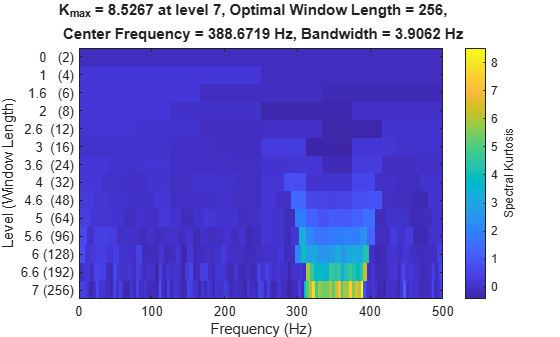The kurtogram shows kurtosis results for a range of window lengths and frequencies. A high kurtosis level corresponds to a high level of nonstationary or non-Gaussian behavior. The peak kurtosis is provided in the text at the top, along with the window length and center frequency associated with it. The bandwidth is a function of the window length.

Explore the effects of lowering the maximum level to 5.

level = 5;
kurtogram(x,fs,level)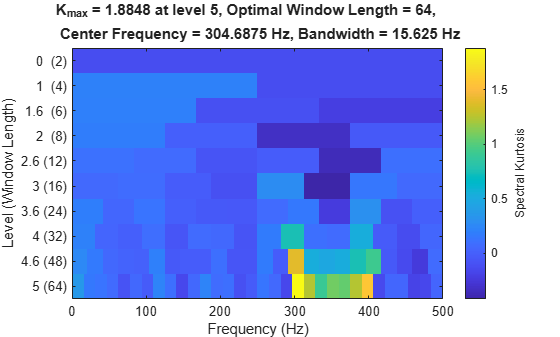The lower resolution is apparent and leads to a lower peak kurtosis value and a displaced center frequency.

Now plot the kurtosis without specifying sample rate or time.

kurtogram(x)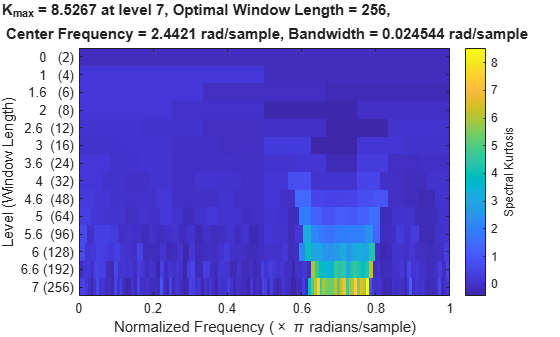The kurtogram is now shown with normalized frequency.

The parameters at the top of the plot provide recommendations for a bandpass filter that could be used to prefilter the data and enhance the differentiation of the nonstationary component. You can also have kurtogram return these values so they can be input more directly into filtering or spectral kurtosis functions.

[kgram,f,w,fc,wc,bw] = kurtogram(x);
wc
wc = 256
fc
fc = 2.4421
bw
bw = 0.0245

These values match the optimal window size, center frequency, and bandwidth of the first plot. kgram is the actual kurtogram matrix, and f and w are the frequency and window-size vectors that accompany it.

The pkurtosis function uses the default pspectrum window size (time resolution). You can specify the window size to use instead. In this example, use the function kurtogram to return an optimal window size and use that result for pkurtosis.

Create a chirp signal with white Gaussian noise.

fs = 1000;
t = 0:1/fs:10;
f1 = 300;
f2 = 400;
x = chirp(t,f1,10,f2)+randn(1,length(t));

Plot the spectral kurtosis with the default window size.

pkurtosis(x,fs)
title('Spectral Kurtosis with Default Window Size')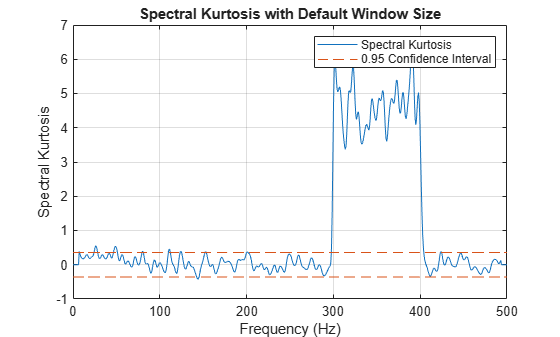Now compute the optimal window size using kurtogram.

kurtogram(x,fs)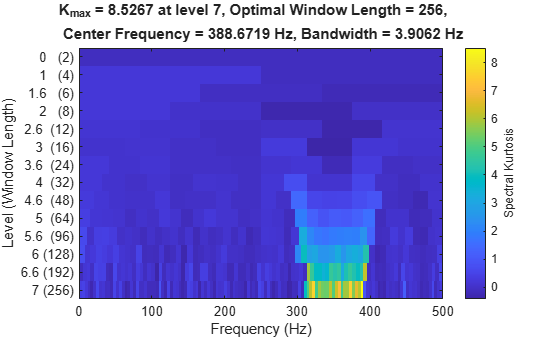The kurtogram plot also illustrates the chirp between 300 and 400 Hz, and shows that the optimum window size is 256. Feed w0 into pkurtosis.

w0 = 256;
pkurtosis(x,fs,w0)
title('Spectral Kurtosis with Optimum Window Size of 256')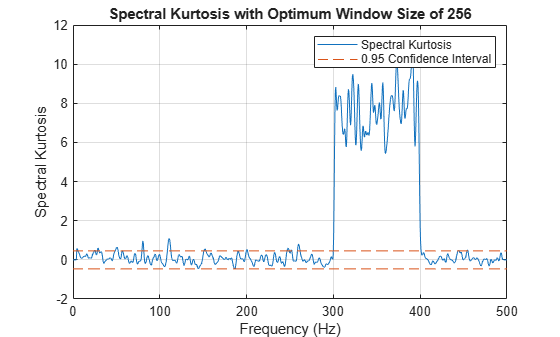The main excursion has higher kurtosis values. The higher values improve the differentiation between stationary and nonstationary components, and enhance your ability to extract the nonstationary component as a feature.

Input Arguments

collapse all

Time-series signal for which kurtogram returns the fast kurtogram, specified as a vector.

Sample rate or sample time, specified as one of the following:

• Positive numeric scalar — Frequency in hertz

• duration scalar — Time interval between consecutive samples of X

• Vector, duration array, or datetime array — Time instant or duration corresponding to each element of x

For an example, see Compute the Kurtogram of a Nonstationary Signal.

When sampx represents a time vector, time samples can be nonuniform, with the pspectrum constraint that the median time interval and the mean time interval must obey:

If you specify sampx as empty, then kurtogram uses normalized frequency. In other words, it assumes an evenly spaced frequency vector spanning [0 π].

Signal timetable from which kurtogram returns the fast kurtogram, specified as a timetable that contains a single variable with a single column. xt must contain increasing, finite row times. If the timetable has missing or duplicate time points, you can fix it using the tips in Clean Timetable with Missing, Duplicate, or Nonuniform Times. xt can be nonuniformly sampled, with the pspectrum constraint that the median time interval and the mean time interval must obey:

Maximum kurtogram level, which drives number of cases to compute, specified as a positive integer. Level drives the frequency window sizes that kurtogram uses, and therefore, the frequency resolution. As frequency resolution, goes up, time resolution goes down. The spectral kurtosis estimate will be poor if either resolution is too low. When you specify level, balance the impact on both time and frequency resolution.

Output Arguments

collapse all

Fast kurtogram, returned as a matrix with dimensions defined by level. kgram has 2*level rows and 3* 2level columns. Each row of the matrix represents the spectral kurtosis results for each element in the frequency vector, and for the window size defined by the position of the row, with respect to the sequence:

$\left[0,1,{\mathrm{log}}_{2}\left(3\right),2,1+{\mathrm{log}}_{2}\left(3\right),3,3+{\mathrm{log}}_{2}\left(3\right),...,n,n+{\mathrm{log}}_{2}\left(3\right),...,level\right],$

where the equivalent window size for a level n is 2n+1 samples.

Frequency vector associated with kgram, returned as a vector. The length of f is equal to the number of columns in kgram.

Window-size vector associated with kgram, returned as a vector. The length of f is equal to the number of columns in kgram.

Frequency of maximal spectral kurtosis value in kgram, returned as a scalar:

• In rad/second, if you have not specified sampx, causing kurtogram to use normalized frequency

• In hertz, if sampx is defined

You can use fc as the central frequency for an optimal bandpass filter that maximizes the kurtosis of the envelope of the filtered signal. Maximizing the envelope kurtosis allows you to more easily extract the resulting impulsive component as a feature.

Window size of maximal spectral kurtosis value in kgram, returned as a scalar in samples. You can use wc to provide the optimal window size for pkurtosis. For an example, see Plot Spectral Kurtosis Using a Customized Window Size.

Suggested bandwidth for optimal bandpass filter, returned as a scalar:

• In rad/second, if you have not specified sampx, causing kurtogram to use normalized frequency

• In hertz, if you have specified sampx

You can use bw to create a filter that maximizes the kurtosis of the envelope of the filtered signal. bw is equal to fx/wc, where fx is the signal sample frequency that kurtogram derives from sampx.